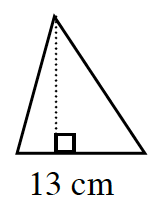### Home > CC1MN > Chapter 10 > Lesson 10.1.3 > Problem10-35

10-35.

If the area of this triangle is $100.75\text{ cm}^2$, what is the height?$\text{Area of a triangle} =\frac{1}{2} (\text{base})(\text{height})$

Substitute all the values that are known.

$\frac{1}{2}(13)h=100.75$

Now solve for h.